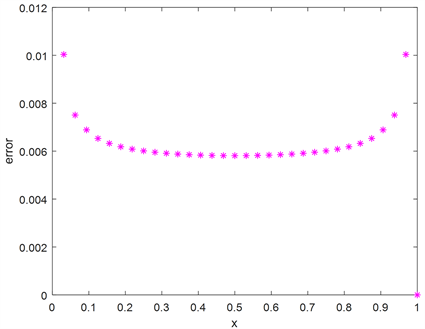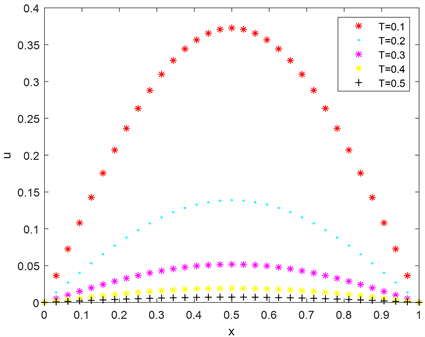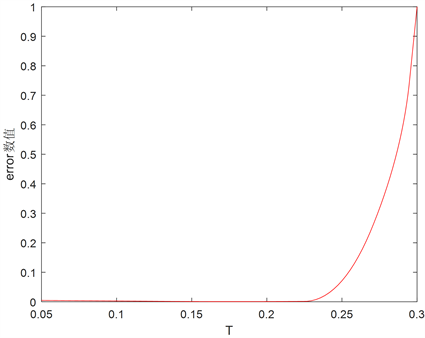﻿ 基于径向基函数的线方法求解热传导方程

# 基于径向基函数的线方法求解热传导方程The Method of Line for Heat Equation Based on Radial Basis Function

Abstract: In this paper, initial and boundary value problem of heat equation is solved by the method of line which is a truly meshless method based on radial basis function. Numerical result shows that the approach is feasible and effective.

1. 引言

$\frac{\partial u\left(x,t\right)}{\partial t}=\alpha \frac{{\partial }^{2}u\left(x,t\right)}{\partial {x}^{2}}+f\left(x\right)$

2. 热传导方程的径向基无网格线方法

$\frac{\partial u\left(x,t\right)}{\partial t}=\alpha \frac{{\partial }^{2}u\left(x,t\right)}{\partial {x}^{2}},\text{ }0\le x\le l,0\le t\le \infty$ (1)

$u\left(x,0\right)=\phi \left(x\right)$ (2)

$u\left(0,t\right)={\mu }_{1}\left(t\right),\text{ }u\left(l,t\right)={\mu }_{2}\left(t\right)$ (3)

${u}^{n}\left(x\right)=\underset{j=1}{\overset{N}{\sum }}{\lambda }_{j}^{n}\left(t\right){\psi }_{j}\left(x\right)={\Psi }^{\text{T}}\left(x\right)\lambda$ (4)

${u}^{n}\left({x}_{i}\right)={u}_{i}$ (5)

$A\lambda =u$ (6)

$u={\left[{u}_{1}\left(t\right),{u}_{2}\left(t\right),\cdots ,{u}_{N}\left(t\right)\right]}^{\text{T}}$ (7)

$\lambda ={\left[{\lambda }_{1}\left(t\right),{\lambda }_{2}\left(t\right),\cdots ,{\lambda }_{N}\left(t\right)\right]}^{\text{T}}$ (8)

$A=\left(\begin{array}{c}{\Psi }^{\text{T}}\left({x}_{\text{1}}\right)\\ {\Psi }^{\text{T}}\left({x}_{\text{2}}\right)\\ ⋮\\ {\Psi }^{\text{T}}\left({x}_{N}\right)\end{array}\right)=\left(\begin{array}{cccc}{\psi }_{1}\left({x}_{1}\right)& {\psi }_{2}\left({x}_{1}\right)& \cdots & {\psi }_{N}\left({x}_{1}\right)\\ {\psi }_{1}\left({x}_{\text{2}}\right)& {\psi }_{2}\left({x}_{\text{2}}\right)& \cdots & {\psi }_{N}\left({x}_{\text{2}}\right)\\ ⋮& ⋮& \ddots & ⋮\\ {\psi }_{1}\left({x}_{N}\right)& {\psi }_{2}\left({x}_{N}\right)& \cdots & {\psi }_{N}\left({x}_{N}\right)\end{array}\right)$ (9)

${u}^{n}\left(x\right)={\Psi }^{\text{T}}\left(x\right){A}^{-1}u=W\left(x\right)u$ (10)

$W\left(x\right)={\Psi }^{\text{T}}\left(x\right){A}^{-1}$ (11)

$\frac{\text{d}{u}_{i}}{\text{d}t}-\alpha {W}_{xx}\left({x}_{i}\right)u=0,\text{ }i=1,2,\cdots ,N$ (12)

${u}_{i}\left(t\right)={u}_{i}$ (13)

${W}_{x}\left({x}_{i}\right)=\left[{W}_{1x}\left({x}_{i}\right){W}_{2x}\left({x}_{i}\right)\cdots {W}_{Nx}\left({x}_{i}\right)\right]$ (14)

${W}_{jxx}\left({x}_{i}\right)=\frac{{\partial }^{2}}{\partial {x}^{2}}{W}_{j}\left({x}_{i}\right),\text{ }j=1,2,\cdots ,N$ (15)

$U={\left[{u}_{1}{u}_{2}\cdots {u}_{N}\right]}^{\text{T}}$ (16)

${W}_{xx}={\left[{W}_{jxx}\left({x}_{i}\right)\right]}_{N\text{×}N}$ (17)

$\frac{\text{d}U}{\text{d}t}-\alpha \left({W}_{xx}U\right)=0$ (18)

$H\left(U\right)=\alpha \left({W}_{xx}U\right)$ (19)

$\frac{\text{d}U}{\text{d}t}=H\left(U\right)$ (20)

${U}^{\text{0}}={\left[\phi \left({x}_{1}\right),\phi \left({x}_{2}\right),\cdots ,\phi \left({x}_{N}\right)\right]}^{\text{T}}$ (21)

$u\left(0,t\right)={\mu }_{1}\left(t\right),\text{ }u\left(l,t\right)={\mu }_{2}\left(t\right)$ (22)

$\left\{\begin{array}{l}{U}^{n+1}={U}^{n}+\frac{\Delta t\left({K}_{1}+2\left({K}_{2}+{K}_{3}\right)+{K}_{4}\right)}{6}\\ {K}_{1}=H\left({U}^{n}\right),{K}_{2}=H\left({U}^{n}+\frac{\Delta t}{2}{K}_{1}\right)\\ {K}_{3}=H\left({U}^{n}+\frac{\Delta t}{2}{K}_{2}\right),{K}_{4}=H\left({U}^{n}+\frac{\Delta t}{2}{K}_{3}\right)\end{array}$ (23)

3. 数值实验

${u}_{z}\left(x,t\right)={\text{e}}^{-{\pi }^{2}t}\mathrm{sin}\text{π}x$ .

$ABE=\underset{i}{\mathrm{max}}|\frac{{u}_{z}\left({x}_{i},{t}_{i}\right)-u\left({x}_{i},{t}_{i}\right)}{{u}_{z}\left({x}_{i},{t}_{i}\right)}|$Figure 1. Comparison of exact and numerical solutionsFigure 2. The distribution of maximum relative errorFigure 3. Numerical solutions at different times

$\text{RAT}=\frac{\Delta t}{{h}^{2}}$Table 1. Maximum relative errors at different timesFigure 4. The changing errors with proportional coefficient RAT

4. 结论Table 2. Maximum relative errors with different nodes

NOTES

*通讯作者。

 姜礼尚. 数学物理方程讲义[M]. 第3版. 北京: 高等教育出版社, 2007.

 李荣华. 偏微分方程数值解法[M]. 北京: 高等教育出版社, 2005.

 冯立伟. 热传导方程几种差分格式的MATLAB数值解法比较[J]. 沈阳化工大学学报, 2011, 25(2): 179-182.

 史策. 热传导方程有限差分法的MATLAB实现[J]. 咸阳师范学院学报, 2009, 24(4): 27-29.

 李玉山. 基于基本解方法求解一维热传导方程移动边界问题[J]. 宝鸡文理学院学报(自科版), 2013, 33(3): 4-9.

 杨继明. 热传导方程初边值问题的谱方法[J]. 湖南工程学院学报(自科版), 2007, 17(2): 71-73.

 Haqa, S., Bibia, N., Tirmizia, S.I.A. and Usmanb, M. (2010) Meshless Method of Lines for the Numerical Solution of Generalized Kuramoto-Sivashinsky Equation. Applied Mathematics & Computation, 217, 2404-2413.
https://doi.org/10.1016/j.amc.2010.07.041

Top